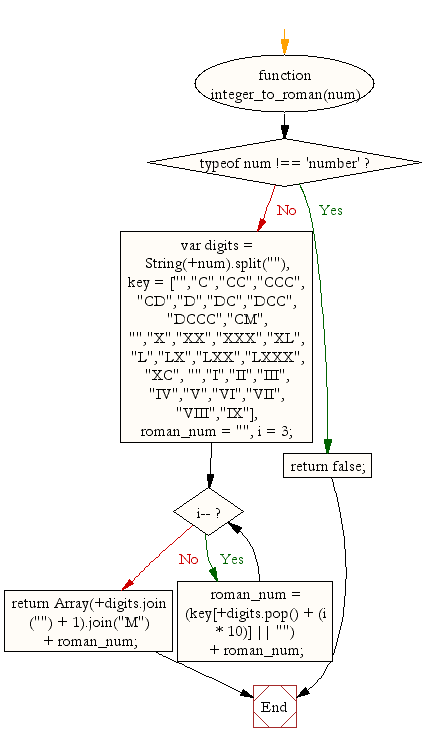# JavaScript: Convert an integer into a Roman Numeral

## JavaScript Math: Exercise-21 with Solution

Write a JavaScript function that Convert an integer into a Roman numeral.

Sample Solution:-

HTML Code:

``````<!DOCTYPE html>
<html>
<head>
<meta charset="utf-8">
<title>Write a JavaScript function that Convert an integer into a Roman Numeral</title>
</head>
<body>

</body>
</html>
```
```

Pictorial Presentation:JavaScript Code:

``````function integer_to_roman(num) {
if (typeof num !== 'number')
return false;

var digits = String(+num).split(""),
key = ["","C","CC","CCC","CD","D","DC","DCC","DCCC","CM",
"","X","XX","XXX","XL","L","LX","LXX","LXXX","XC",
"","I","II","III","IV","V","VI","VII","VIII","IX"],
roman_num = "",
i = 3;
while (i--)
roman_num = (key[+digits.pop() + (i * 10)] || "") + roman_num;
return Array(+digits.join("") + 1).join("M") + roman_num;
}

console.log(integer_to_roman(27));
```
```

Sample Output:

```XXVII
```

Flowchart:Live Demo:

See the Pen javascript-math-exercise-21 by w3resource (@w3resource) on CodePen.

Improve this sample solution and post your code through Disqus

What is the difficulty level of this exercise?

﻿

## JavaScript: Tips of the Day

Returns every element that exists in any of the two arrays once, using a provided comparator function

Example:

```const tips_unionWith = (a, b, comp) =>
Array.from(new Set([...a, ...b.filter(x => a.findIndex(y => comp(x, y)) === -1)]));
?
console.log(tips_unionWith([1, 2.5, 3.5, 4, 0], [4.5, 3, 0, 3.9], (a, b) => Math.round(a) === Math.round(b)));
```

Output:

```[1,2.5,3.5,4,0,4.5]
```